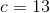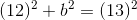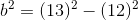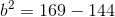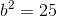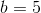# High School Math : How to find the length of the side of a right triangle

## Example Questions

### Example Question #4 : How To Find The Length Of The Side Of A Right Triangle

A right triangle with a base of 12 and hypotenuse of 15 is shown below. Find x.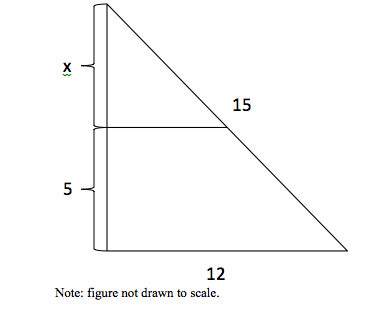4.5

3.5

4

5

5.5

4

Explanation:

Using the Pythagorean Theorem, the height of the right triangle is found to be = √(〖15〗–〖12〗2) = 9, so x=9 – 5=4

### Example Question #5 : How To Find The Length Of The Side Of A Right Triangle

A right triangle has sides of 36 and 39(hypotenuse).  Find the length of the third side

42

15

33

33√2

12 √6

15

Explanation:

use the pythagorean theorem:

a2 + b2 = c2  ; a and b are sides, c is the hypotenuse

a2 + 1296 = 1521

a2 = 225

a = 15

### Example Question #6 : How To Find The Length Of The Side Of A Right Triangle

Bob the Helicopter is at 30,000 ft. above sea level, and as viewed on a map his airport is 40,000 ft. away. If Bob travels in a straight line to his airport at 250 feet per second, how many minutes will it take him to arrive?

2 hours and 30 minutes

3 minutes and 20 seconds

4 hours and 0 minutes

3 minutes and 50 seconds

1 hour and 45 minutes

3 minutes and 20 seconds

Explanation:

Draw a right triangle with a height of 30,000 ft. and a base of 40,000 ft. The hypotenuse, or distance travelled, is then 50,000ft using the Pythagorean Theorem. Then dividing distance by speed will give us time, which is 200 seconds, or 3 minutes and 20 seconds.

### Example Question #7 : How To Find The Length Of The Side Of A Right Triangle

A right triangle has two sides, 9 and x, and a hypotenuse of 15. What is x?

13

11

10

14

12

12

Explanation:

We can use the Pythagorean Theorem to solve for x.

92 + x2 = 152

81 + x2 = 225

x2 = 144

x = 12

### Example Question #8 : How To Find The Length Of The Side Of A Right Triangle

The area of a right traingle is 42. One of the legs has a length of 12. What is the length of the other leg?Explanation: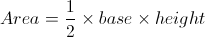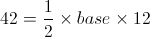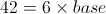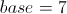### Example Question #1 : How To Find The Length Of The Side Of A Right Triangle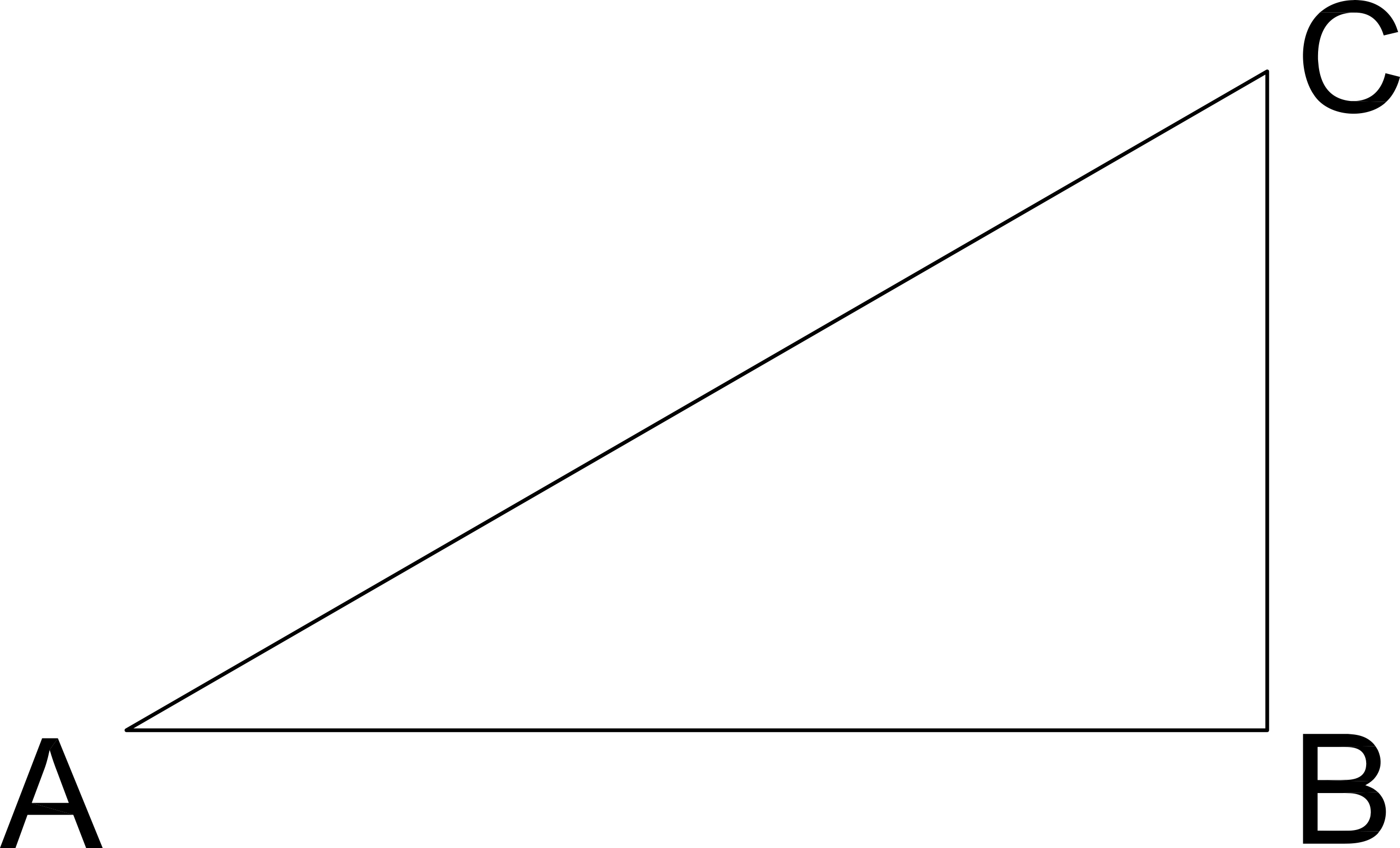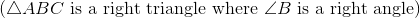If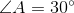and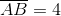, what is the length of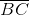?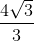Explanation:

AB is the leg adjacent to Angle A and BC is the leg opposite Angle A.

Since we have a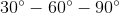triangle, the opposites sides of those angles will be in the ratio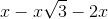.

Here, we know the side opposite the sixty degree angle. Thus, we can set that value equal to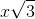.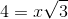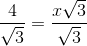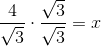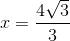which also means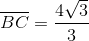### Example Question #9 : How To Find The Length Of The Side Of A Right Triangle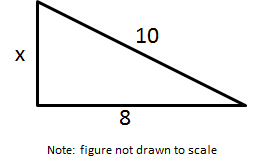Solve for x.

6

2

7

12

6

Explanation:

Use the Pythagorean Theorem. Let a = 8 and = 10 (because it is the hypotenuse)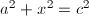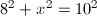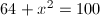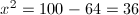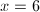### Example Question #11 : How To Find The Length Of The Side Of A Right Triangle

Solve for.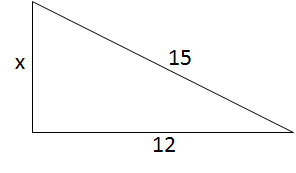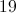Explanation:

Use the Pythagorean Theorem to solve for the missing side of the right triangle.In this triangle,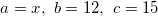.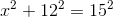Now we can solve for.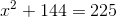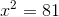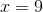### Example Question #12 : How To Find The Length Of The Side Of A Right Triangle

Solve for.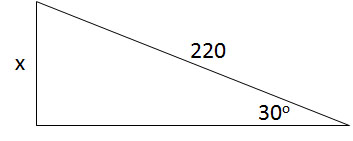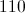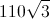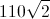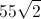Explanation:

This image depicts a 30-60-90 right triangle. The length of the side opposite the smallest angle is half the length of the hypotenuse.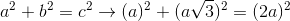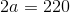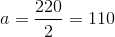### Example Question #13 : How To Find The Length Of The Side Of A Right Triangle

Given a right triangle, solve for the missing leg if one leg is 12 and the hypotenuse is 13.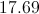Explanation:

Since the traingle is a right traingle, we can use the Pythagorean Theorem to solve for the missing leg: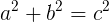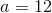and the hypotenuse